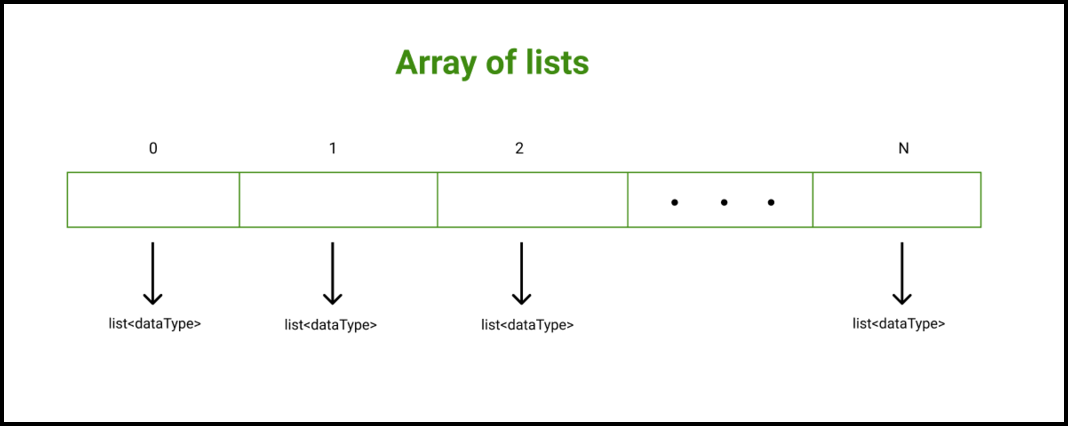# Array of list in C++ with Examples

• Difficulty Level : Basic
• Last Updated : 24 Jan, 2022

What is an array?

An array in any programming language is a data structure that is used to store elements or data items of similar data types at contiguous memory locations and elements can be accessed randomly using indices of an array. Arrays are efficient when we want to store a large number of elements that too of similar data types.

What is a list?

In C++, a list is a sequence container that allows non-contiguous memory allocation. If we compare a vector with a list, then a list has slow traversal as compared to a vector but once a position has been found, insertion and deletion are quick. Generally, a list in C++ is a doubly-linked list.

Functions used with List:

• front(): Returns the value of the first element in the list.
• back(): Returns the value of the last element in the list.
• push_front(x): Adds a new element ‘x’ at the beginning of the list.
• push_back(x): Adds a new element ‘x’ at the end of the list.
• pop_front(): Removes the first element of the list, and reduces the size of the list by 1.
• pop_back(): Removes the last element of the list, and reduces the size of the list by 1.

Array of lists

C++ allows us a facility to create an array of lists. An array of lists is an array in which each element is a list on its own.

Syntax:

list<dataType> myContainer[N];

Here,
N: The size of the array of the lists.
dataType: A dataType. It signifies that each list can store elements of this data type only.Example 1: Below is the C++ program to implement an array of lists.

## C++

 `// C++ program to demonstrate the``// working of array of lists in C++``#include ``using` `namespace` `std;`` ` `// Function to print list elements``// specified at the index, "index"``void` `print(list<``int``>& mylist,``           ``int` `index)``{``    ``cout << ``"The list elements stored at the index "` `<< ``             ``index << ``": \n"``;`` ` `    ``// Each element of the list is a pair on ``    ``// its own``    ``for` `(``auto` `element : mylist) ``    ``{``        ``// Each element of the list is a pair ``        ``// on its own``        ``cout << element << ``'\n'``;``    ``}``    ``cout << ``'\n'``;``}`` ` `// Function to iterate over all the array``void` `print(list<``int``>* myContainer, ``int` `n)``{``    ``cout << ``"myContainer elements:\n\n"``;`` ` `    ``// Iterating over myContainer elements``    ``// Each element is a list on its own``    ``for` `(``int` `i = 0; i < n; i++) ``    ``{``        ``print(myContainer[i], i);``    ``}``}`` ` `// Driver code``int` `main()``{``    ``// Declaring an array of lists``    ``// In list each element is of type int``    ``list<``int``> myContainer;`` ` `    ``// listing values to the list stored``    ``// at the index 0``    ``// 15 <-> 5 <-> 10 <-> 20``    ``myContainer.push_front(5);``    ``myContainer.push_back(10);``    ``myContainer.push_front(15);``    ``myContainer.push_back(20);`` ` `    ``// listing values to the list stored``    ``// at the index 1``    ``// 40 <-> 30 <-> 35 <-> 45``    ``myContainer.push_front(30);``    ``myContainer.push_back(35);``    ``myContainer.push_front(40);``    ``myContainer.push_back(45);`` ` `    ``// listing values to the list stored``    ``// at the index 2``    ``// 60 <-> 50 <-> 55 <-> 65``    ``myContainer.push_front(50);``    ``myContainer.push_back(55);``    ``myContainer.push_front(60);``    ``myContainer.push_back(65);`` ` `    ``// Calling print function to iterate``    ``// over myContainer elements``    ``print(myContainer, 3);`` ` `    ``return` `0;``}`
="code-output">Output

myContainer elements:

The list elements stored at the index 0:
15
5
10
20

The list elements stored at the index 1:
40
30
35
45

The list elements stored at the index 2:
60
50
55
65

Example 2: Below is the C++ program to implement an array of lists.

## C++

 `// C++ program to demonstrate the``// working of array of lists in C++``#include ``using` `namespace` `std;`` ` `// Function to print list elements``// specified at the index, "index"``void` `print(list& mylist,``           ``int` `index)``{``    ``cout << ``"The list elements stored at the index "` `<< ``             ``index << ``": \n"``;`` ` `    ``// Each element of the list is a pair ``    ``// on its own``    ``for` `(``auto` `element : mylist) ``    ``{``        ``// Each element of the list is a pair ``        ``// on its own``        ``cout << element << ``'\n'``;``    ``}``    ``cout << ``'\n'``;``}`` ` `// Function to iterate over all the array``void` `print(list* myContainer, ``int` `n)``{``    ``cout << ``"myContainer elements:\n\n"``;`` ` `    ``// Iterating over myContainer elements``    ``// Each element is a list on its own``    ``for` `(``int` `i = 0; i < n; i++) ``    ``{``        ``print(myContainer[i], i);``    ``}``}`` ` `// Driver code``int` `main()``{``    ``// Declaring an array of lists``    ``// In list each element is of type string``    ``list myContainer;`` ` `    ``// listing values to the list stored``    ``// at the index 0``    ``// "GeeksforGeeks" <-> "C++" <-> ``    ``// "Python" <-> "C"``    ``myContainer.push_front(``"C++"``);``    ``myContainer.push_back(``"Python"``);``    ``myContainer.push_front(``"GeeksforGeeks"``);``    ``myContainer.push_back(``"C"``);`` ` `    ``// listing values to the list stored``    ``// at the index 1``    ``// "Nainwal" <-> "Java" <-> "C#" <-> "GFG"``    ``myContainer.push_front(``"Java"``);``    ``myContainer.push_back(``"C#"``);``    ``myContainer.push_front(``"Nainwal"``);``    ``myContainer.push_back(``"GFG"``);`` ` `    ``// listing values to the list stored``    ``// at the index 2``    ``// "HTML" <-> "Swift" <-> "R" <-> "CSS"``    ``myContainer.push_front(``"Swift"``);``    ``myContainer.push_back(``"R"``);``    ``myContainer.push_front(``"HTML"``);``    ``myContainer.push_back(``"CSS"``);`` ` `    ``// Calling print function to iterate``    ``// over myContainer elements``    ``print(myContainer, 3);`` ` `    ``return` `0;``}`
Output

myContainer elements:

The list elements stored at the index 0:
GeeksforGeeks
C++
Python
C

The list elements stored at the index 1:
Nainwal
Java
C#
GFG

The list elements stored at the index 2:
HTML
Swift
R
CSS

My Personal Notes arrow_drop_up